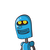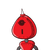# (b) Two crore ninety lakh fifty five thousand eight hundred.(c) Seven crore sixty thousand fifty five.G1-You hav

(b) Two crore ninety lakh fifty five thousand eight hundred.
(c) Seven crore sixty thousand fifty five.
G
1-You have the following digits 4,5,6, 0, 7 and 8. Using them, make
five numbers each with 6 digits.
(a) Put commas for easy reading.
(b) Arrange them in ascending and descending order.
Take the digits 4,5,6,7,8 and 9. Make any three numbers each with
3. From the digits 3,0 and 4, make five numbers each with 6 digits. Use
commas.
w han lingkungan
Sale State Board of Sthqgl Educatia​

### 2 thoughts on “(b) Two crore ninety lakh fifty five thousand eight hundred.<br />(c) Seven crore sixty thousand fifty five.<br />G<br />1-You hav”

1.G. 456078, 560784, 607845, 780546, 876540

2.→ Two crore ninety lakh fifty five thousand eight hundred = 2,90,55,800 .

→ Seven crore sixty thousand fifty five = 7,00,60,055 .

now, given digits are :- 4,5,6, 0, 7 and 8.

so,

→ 5 numbers each with 6 digits in ascending order = 4,05,678 < 4,50,678 < 4,56,078 < 4,57,608 < 4,58,706 .

→ 5 numbers each with 6 digits in descending order =

8,76,540 > 7,86,405 > 6,87,540 > 6, 80,745 > 5,76,840 .

now, given digits are :- 4,5,6,7,8 and 9 .

→ 3 numbers each with 6 digits = 4,56,789 and 5,49,786 and 9,56,784 .

now, given digits are :- 3, 0 and 4 .

→ 5 numbers each with 6 digits = 3,04,304 and 3,00,404 and 4,00,03 and 4,33,000 and 3,40,403 .